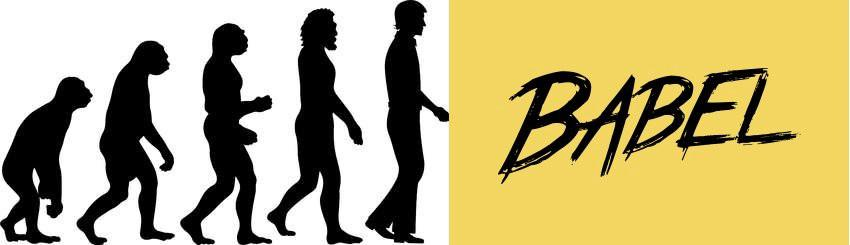## 零、前言

``````// Shape 类
function Shape(id, x, y) {
this.id = id;
this.setLocation(x, y);
}

// 设置坐标的原型方法
Shape.prototype.setLocation = function(x, y) {
this.x = x;
this.y = y;
};
``````

``````class Shape {
constructor(id, x, y) { // 构造函数语法糖
this.id = id;
this.setLocation(x, y);
}

setLocation(x, y) { // 原型方法
this.x = x;
this.y = y;
}
}
``````

"use strict";var _createClass=function(){function a(a,b){for(var c,d=0;d<b.length;d++)c=b[d],c.enumerable=c.enumerable||!1,c.configurable=!0,"value"in c&&(c.writable=!0),Object.defineProperty(a,c.key,c)}return function(b,c,d){return c&&a(b.prototype,c),d&&a(b,d),b}}();function _classCallCheck(a,b){if(!(a instanceof b))throw new TypeError("Cannot call a class as a function")}var Shape=function(){function a(b,c,d){_classCallCheck(this,a),this.id=b,this.setLocation(c,d)}return _createClass(a,[{key:"setLocation",value:function c(a,b){this.x=a,this.y=b}}]),a}();

Babel 仅仅是把我们定义的 Shape 还原成一个 ES5 函数与对应的原型方法么？

## 一、揭秘

``````class Shape {}
``````

``````"use strict";

class Shape {}
``````

``````"use strict";

var Shape = class Shape {};
``````

``````"use strict";

function _classCallCheck(instance, Constructor) { if (!(instance instanceof Constructor)) { throw new TypeError("Cannot call a class as a function"); } }

var Shape = function Shape() {
_classCallCheck(this, Shape);
};
``````

``````"use strict";

function _classCallCheck(instance, Constructor) { if (!(instance instanceof Constructor)) throw new TypeError("Cannot call a class as a function"); }

var Shape = function Shape() {
_classCallCheck(this, Shape);
};
``````

``````"use strict";

function _classCallCheck(a, b) { if (!(a instanceof b)) throw new TypeError("Cannot call a class as a function"); }

var Shape = function a() {
_classCallCheck(this, a);
};
``````

"use strict";function _classCallCheck(a,b){if(!(a instanceof b))throw new TypeError("Cannot call a class as a function")}var Shape=function a(){_classCallCheck(this,a)};

## 二、再说一些

``````class Shape {
render() {
console.log("Hi");
}
}
``````

var _createClass = function () { function a(a, b) { for (var c, d = 0; d < b.length; d++) c = b[d], c.enumerable = c.enumerable || !1, c.configurable = !0, "value" in c && (c.writable = !0), Object.defineProperty(a, c.key, c); } return function (b, c, d) { return c && a(b.prototype, c), d && a(b, d), b; }; }();

``````var _createClass = function() {
function defineProperties(target, props) {
for (var i = 0; i < props.length; i++) {
var descriptor = props[i];
descriptor.enumerable = descriptor.enumerable || false;
descriptor.configurable = true;
if ("value" in descriptor) descriptor.writable = true;
Object.defineProperty(target, descriptor.key, descriptor);
}
}
return function(Constructor, protoProps, staticProps) {
if (protoProps) defineProperties(Constructor.prototype, protoProps);
if (staticProps) defineProperties(Constructor, staticProps);
return Constructor;
};
} ();
``````

``````_createClass(Shape, [{
key: "render",
value: function render() {
console.log("Hi");
}
}]);
``````

T.J. Crowder 在 How does Babel.js create compile a class declaration into ES2015? 中谈到 Babel 是如何将 class 转化为 ES5 兼容代码时谈到了几点，大意为：

• `constructor` 会成为构造方法数；
• 所有非构造方法、非静态方法会成为原型方法；
• 静态方法会被赋值到构造函数的属性上，其他属性保持不变；
• 派生构造函数上的原型属性是通过 `Object.create(Base.prototype)` 构造的对象，而不是 `new Base()`
• `constructor` 调用构造器基类是第一步操作；
• ES5 中对应 `super` 方法的写法是 `Base.prototype.baseMethod.call(this);`，这种操作不仅繁琐而且容易出错；